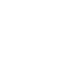# SAT Question of the Day (ACT Too!): Dec. 12, 2013

If you are reading this in an email you received from me, do not click the link to sat.collegeboard.org below. Use the link to my website that is farther down on the email.

If you are seeing this in my blog, do the SAT Question of the Day by clicking on this link:

http://sat.collegeboard.org/practice/sat-question-of-the-day?questionId=20131212&oq=1 (This link takes you to today’s question. If you use my archive, you will see the question related to my SAT explanation for that date.)

The answer is C.  The probability that something will happen is a fraction that has the “number of correct ways something can happen” in the numerator and the “number of possible ways something can happen” in the denominator.  Because AB is 2 and BC is 3, there are a total of 5 possibilities.  (Think of the line as 5 inches long with two inches from A to B and three inches from B to C.)  The total is 5, the denominator.  There are 3 “correct” inches (from B to C) for the point that is chosen at random, the numerator.  Therefore, the answer is 3/5.

This question is a good reminder of why practice is important.  I suspect you’ve been taught probability in school.  However, the test writers are always sitting around trying to think of new ways to ask questions like you’ve never seen before.  I bet you’ve never seen a probability question about points on a line like this one.  If you’ll practice, you’ll see more examples of questions that are like the situations that are important to the test writers.  That will help eliminate surprises on test day which would be a good thing!

Let’s see what the ACT folks have for us today.

Wow!  A new question.  That’s wonderful.  I guess the recent bad weather in Iowa forced the test writers into their offices so that they could program a new question for us.

The answer is F.   This is an unusual ACT question because they give you the formula you need to answer the question.  Starting with the formula, you just need to plug the numbers they give you into it and turn the math crank.  Take a look at the test writer’s explanation and you’ll see one way to do the math.  I did it a different way and did the calculation in my head rather than do algebra and use a calculator.  Doing so was very fast and accurate, but it will take you some practice to master the strategy.

Look at the equation a different way.  The altitude times the average of the lengths of the two bases is the area!  Did you see how the equation includes 1/2 times (b1 + b2)?  That is the same as dividing (b1 + b2) by 2 which is the average of the length of the two bases.  The area is 55; so, the average of the two bases is 11 because the altitude is 5.  One base is 13 and that means the other base has to be 9 in order for their average to be 11.  All this can be done in your head very quickly.

Do you know how I figured out the unknown base had to be 9 without calculating an average the normal way?  The average of two numbers has to be the “balancing point” between them.  13 is two greater than 11; therefore, the other number has to be 2 less than 11 which is 9, the right answer.  For further information about this wonderful Wizardly strategy, take a look at the math tip video on the home page of my web site.  There’s also more about it on my free website and on Video #8.

I hope the weather in your area isn’t too bad.  Many of you are probably getting extra days off school.  Make good use of them.  Catch up on your studies or even get a little ahead.  Put a fire in the fireplace and drink some hot chocolate for me.  I’m in Florida and it has been above 80 degrees every day this month.  (Yes, I’m rubbing it in!)

The SAT & ACT Wizard